# 16 Results

View
Selected filters:
• MCCRS.Math.Content.6.NS.C.6
Remix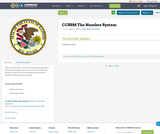Conditional Remix & Share Permitted
CC BY-SA
Rating

This resource links to both the Fractions progression document published by the Common Core Writing Teams in June 2011 and the Module posted on the Illustrative Mathematics website.

Material Type:
Assessment
Homework/Assignment
Teaching/Learning Strategy
Author:
ISBE Math Specialists
08/08/2019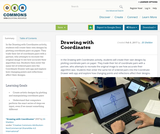Conditional Remix & Share Permitted
CC BY-NC-SA
Rating

In the Drawing with Coordinates activity, students will create their own designs by plotting coordinate pairs on paper. They trade their list of coordinate pairs with a partner, who attempts to recreate the original image to see how accurate their algorithm was. Students then enter the same list of ordered pairs into the Coordinate Drawer web app and explore how changing points and reflections affect their designs.

Subject:
Mathematics
Material Type:
Activity/Lab
Author:
Eli Sheldon
02/09/2017Educational Use
Rating

Students learn about the Foucault pendulum an engineering tool used to demonstrate and measure the Earth's rotation. Student groups create small experimental versions, each comprised of a pendulum and a video camera mounted on a rotating platform actuated by a LEGO MINDSTORMS(TM) NXT motor. When the platform is fixed, the pendulum motion forms a line, as observed in the recorded video. When the rotating, the pendulum's motion is observed as a set of spirals with a common center. Observing the patterns that the pendulum bob makes when the platform is rotating provides insight as to how a full-size Foucault pendulum operates. It helps students understand some of the physical phenomena induced by the Earth's rotation, as well as the tricky concept of how the perception of movement varies, depending on one's frame of reference.

Subject:
Engineering
Physics
Material Type:
Activity/Lab
Provider:
TeachEngineering
Provider Set:
TeachEngineering
Author:
Jeffrey Laut
Violet Mwaffo
09/18/2014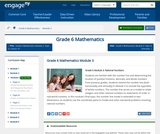Conditional Remix & Share Permitted
CC BY-NC-SA
Rating

Students are familiar with the number line and determining the location of positive fractions, decimals, and whole numbers from previous grades. Students extend the number line (both horizontally and vertically) in Module 3 to include the opposites of whole numbers. The number line serves as a model to relate integers and other rational numbers to statements of order in real-world contexts. In this module's final topic, the number line model is extended to two-dimensions, as students use the coordinate plane to model and solve real-world problems involving rational numbers.

Subject:
Numbers and Operations
Material Type:
Module
Provider:
New York State Education Department
Provider Set:
EngageNY
10/20/2013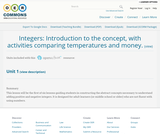Unrestricted Use
CC BY
Rating

This lesson will be the first of six lessons guiding students in constructing the abstract concepts necessary to understand adding positive and negative integers.
It is designed for adult learners (or middle school or older) who are not fluent with using numbers.

Subject:
Mathematics
Material Type:
Lesson Plan
Author:
Susan Jones
06/08/2018Conditional Remix & Share Permitted
CC BY-NC
Rating

Subject:
Mathematics
Material Type:
Full Course
Provider:
Pearson
10/06/2016Rating

Rational Numbers

Type of Unit: Concept

Prior Knowledge

Students should be able to:

Solve problems with positive rational numbers.
Plot positive rational numbers on a number line.
Understand the equal sign.
Use the greater than and less than symbols with positive numbers (not variables) and understand their relative positions on a number line.
Recognize the first quadrant of the coordinate plane.

Lesson Flow

The first part of this unit builds on the prerequisite skills needed to develop the concept of negative numbers, the opposites of numbers, and absolute value. The unit starts with a real-world application that uses negative numbers so that students understand the need for them. The unit then introduces the idea of the opposite of a number and its absolute value and compares the difference in the definitions. The number line and positions of numbers on the number line is at the heart of the unit, including comparing positions with less than or greater than symbols.

The second part of the unit deals with the coordinate plane and extends student knowledge to all four quadrants. Students graph geometric figures on the coordinate plane and do initial calculations of distances that are a straight line. Students conclude the unit by investigating the reflections of figures across the x- and y-axes on the coordinate plane.

Subject:
Mathematics
Numbers and Operations
Material Type:
Unit of Study
Provider:
PearsonConditional Remix & Share Permitted
CC BY-NC
Rating

Students revise their work on the Self Check based on feedback from the teacher and their peers.Key ConceptsConcepts from previous lessons are integrated into this assessment task: integers, absolute value, and comparing numbers. Students apply their knowledge, review their work, and make revisions based on feedback from the teacher and their peers. This process creates a deeper understanding of the concepts.Goals and Learning ObjectivesApply your knowledge of integers, absolute value, and comparing numbers to solve problems.Track and review your choice of strategy when problem solving.

Subject:
Numbers and Operations
Material Type:
Lesson Plan
Provider:
Pearson
09/21/2015Conditional Remix & Share Permitted
CC BY-NC
Rating

Students draw a figure on the coordinate plane that matches a written description.Key ConceptsOrdered pairs name locations on the coordinate plane. The first coordinate tells how many units to go left or right of the origin (0,0) along the x-axis. The second coordinate tells how many units to go up or down from the origin along the y-axis.Goals and Learning ObjectivesDraw a figure that matches a description of a figure on the coordinate plane.Give coordinates of points on the coordinate plane.Write descriptions of figures on the coordinate plane.

Subject:
Numbers and Operations
Material Type:
Lesson Plan
Provider:
Pearson
09/21/2015Conditional Remix & Share Permitted
CC BY-NC
Rating

Students identify whether an inequality statement is true or false using a number line to support their reasoning.Key ConceptsThe meaning of mThe meaning of n > m is that n is located to the right of m on a number line. The inequality statement n > m is read “n is greater than m.”To decide on the order of two numbers m and n, locate the numbers on a number line. If m is to the left of n, then m < n. If m is to the right of n, then m > n.Goals and Learning ObjectivesState whether an inequality is true or false.Use a number line to prove that an inequality is true or false.

Subject:
Numbers and Operations
Material Type:
Lesson Plan
Provider:
Pearson
09/21/2015Conditional Remix & Share Permitted
CC BY-NC
Rating

Students watch a dot get tossed from one number on a number line to the opposite of the number. Students predict where the dot will land each time based on its starting location.Key ConceptsThe opposite of a number is the same distance from 0 as the number itself, but on the other side of 0 on a number line.In the diagram, m is the opposite of n, and n is the opposite of m. The distance from m to 0 is d, and the distance from n to 0 is d; this distance to 0 is the same for both n and m. The absolute value of a number is its distance from 0 on a number line.Positive numbers are numbers that are greater than 0.Negative numbers are numbers that are less than 0.The opposite of a positive number is negative, and the opposite of a negative number is positive.Since the opposite of 0 is 0 (which is neither positive nor negative), then 0 = 0. The number 0 is the only number that is its own opposite.Whole numbers and the opposites of those numbers are all integers.Rational numbers are numbers that can be expressed as ab, where a and b are integers and b ≠ 0.Goals and Learning ObjectivesIdentify a number and its oppositeLocate the opposite of a number on a number lineDefine the opposite of a number, negative numbers, rational numbers, and integers

Subject:
Numbers and Operations
Material Type:
Lesson Plan
Provider:
Pearson
09/21/2015Conditional Remix & Share Permitted
CC BY-NC
Rating

Students analyze whether given statements are possible or impossible using their definitions of absolute value and the opposite of a number. If the statements are possible, students give an example of a pair of numbers that fit the statement. If the statements are impossible, students explain why.Key ConceptsA number and the opposite of the number always have the same absolute value.In general, taking the opposite of n changes the sign of n. For example, the opposite of 3 is −3.In general, taking the absolute value of n gives a number |n|, which is always positive. For example, |3| = 3 and |−3| = 3.Since the opposite of 0 is 0 (which is neither positive nor negative), therefore −0 = 0. The number 0 is the only number which is its own opposite.Goals and Learning ObjectivesFind pairs of numbers that satisfy different statements about absolute values and/or the opposites of numbers.State when it is impossible to find a pair of numbers that satisfies the statement and explain why.

Subject:
Numbers and Operations
Material Type:
Lesson Plan
Provider:
Pearson
09/21/2015Conditional Remix & Share Permitted
CC BY-NC
Rating

Students reflect a figure across one of the axes on the coordinate plane and name the vertices of the reflection. As they are working, students look for and make use of structure to identify a convention for naming the coordinates of the reflected figure.Key ConceptsWhen point (m,n) is reflected across the y-axis, the reflected point is (−m,n).When point (m,n) is reflected across the x-axis, the reflected point is (m,−n).When point (m,n) is reflected across the origin (0,0), the reflected point is (−m,−n).Goals and Learning ObjectivesReflect a figure across one of the axes on the coordinate plane.Name the vertices of the reflected figure.Discern a pattern in the coordinates of the reflected figure.

Subject:
Numbers and Operations
Material Type:
Lesson Plan
Provider:
Pearson
09/21/2015Conditional Remix & Share Permitted
CC BY-NC
Rating

Students answer questions about low temperatures recorded in Barrow, Alaska, to understand when to use negative numbers and when to use the absolute values of numbers.Key ConceptsThe absolute value of a number is its distance from 0 on a number line.The absolute value of a number n is written |n| and is read as “the absolute value of n.”A number and the opposite of the number always have the same absolute value. As shown in the diagram, |3| = 3 and |−3| = 3.In general, taking the opposite of n changes the sign of n. For example, the opposite of 3 is –3.In general, taking the absolute value of n gives a number, |n|, that is always positive unless n = 0. For example, |3| = 3 and |−3| = 3.The absolute value of 0 is 0, which is neither positive nor negative: |0| = 0.Goals and Learning ObjectivesUnderstand when to talk about a number as negative and when to talk about the absolute value of a number.Locate the absolute value of a and the absolute value of b on a number line that shows the location of a and b in different places in relation to 0.

Subject:
Numbers and Operations
Material Type:
Lesson Plan
Provider:
Pearson
09/21/2015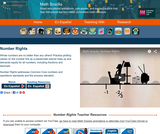Rating

Whole numbers are no better than any others! Practice plotting values on the number line as a passionate activist rises up and demands equity for all numbers, including fractions and decimals.

Subject:
Mathematics
Material Type:
Lecture
Provider:
Learning Games Lab
Author:
NMSU Learning Games Lab
07/15/2015Rating

Navigate the number line while diving amidst shipwrecks and sunken ruins. Will you find a pearl, or an old boot? Watch out for the electric eel! Pearl Diver teaches properties of numbers, how to plot numbers, how to visualize quantity on the number line, how to order numbers, and how to use the number line as a visual model for mathematical operations.

Subject:
Mathematics
Material Type:
Game
Interactive
Provider:
Learning Games Lab
Author:
NMSU Learning Games Lab